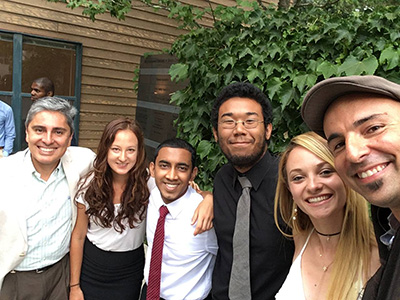/ / / / /

# MSRI UP 2017 Projects

 Home Research Topic People Colloquia Research Projects Pictures

Counting the roots of a polynomial modulo p^2

Students: Trajan Hammonds, Jeremy Johnson, Angela Patini
Mentors: J. Maurice Rojas + Robert Walker

Abstract:

Until recently, the only known method of finding the roots of polynomials over prime power rings, other than fields, was brute force. One reason for this is the lack of a division algorithm, thus obstructing the use of greatest common divisors. Suppose f is any nonzero univariate polynomial of degree d in Z/p^nZ[x]. For the case n=2, we prove a new efficient algorithm to count the roots in Z/p^2Z within time polynomial in d + log p. The key idea is to partition the roots via their image under reduction modulo p, and then carefully use Hensel's Lemma. This builds upon recent work of Cheng, Gao, Rojas, and Wan.On the maximal number of roots of a trinomial over a prime field

Students: Jeshu Dastidar, Viviana Peña Márquez, Ryan Pugh
Mentors: J. Maurice Rojas + Megan Ly

Abstract:

Canetti, Friedlander, et al. (2002) studied the randomness of powers over finite fields and along the way derived an analogue of Descartes’™ rule over the finite field F_q with q elements: They showed that the number of roots of any univariate t-nomial, with exponents {0,a_2,...,a_t} and the differences a_i-a_j all relatively prime to q-1, is O(q^{(t-2)/(t-1)}). The correct optimal bounds remain a mystery for prime fields, even in the case of polynomials with three terms. Following the work of Kelley (2016), we seek to prove the conjecture that the number of roots in F_p of a trinomial with a linear middle term is always O(log p). We expand current evidence by using a supercomputer to determine the  number of roots of these trinomials for 139,571 < p 191,491. We also prove that the search can be restricted to trinomials with a middle linear term when p-1 has less than three distinct prime factors.Applying discriminant chambers to structured polynomials

Students: Amy Adair, Alexander Mendez, Diane Tchuindjo
Mentors: J. Maurice Rojas + Robert Walker

Abstract:

One way to prove the algorithmic hardness of a function is to consider its circuit complexity: the minimal size of a circuit computing the function. Recent work in circuit complexity has revealed that it is enough to restrict to depth-4 circuits, inspiring the study of certain structured polynomials called sum-of-products-of-sparse (SPS) polynomials. In particular, showing sufficiently good upper bounds for the number of real roots of SPS polynomials would imply the hardness of the permanent. If f and g are univariate polynomials with real coefficients and t terms, then does fg-1 have a number of real roots that grows at most linearly in t? We consider the first non-trivial approach towards such a bound via A-discriminants, leading to interesting questions involving sparse polynomial systems.Using lower binomials to approximate roots of trinomials

Students: Esteban Madrigal, Carlos Osco Huaricapcha, Harold Jiménez Polo
Mentors: J. Maurice Rojas + Anastasia Chavez

Abstract:

Given a univariate trinomial f in R[x], we analyze the Archimedean Newton polytope of f and the corresponding lower binomials. The roots of these lower binomials conjecturally provide high quality approximations of the roots of f. We implement Smale's alpha-criterion to analyze whether our approximations converge quickly under Newton iteration. We know that under certain conditions every root of a lower binomial is an approximate root of a trinomial. We expect to determine when at least one root of a lower binomial is an approximate root. Moreover, for roots that are not approximate, we examine when Newton's method yields approximate roots.Topology of positive zero sets of bivariate pentanomials

Students: Malachi Alexander, Ashley De Luna, Christian McRoberts
Mentors: J. Maurice Rojas + Megan Ly

Abstract:

A fundamental problem in many applications is determining the real solutions of a polynomial. In recent years, the A-discriminant variety of Gelfand, Kapranov and Zelevinsky has proved to be a valuable tool. Its complement over the real numbers defines regions of coefficient values called chambers. We implement an algorithm in MATLAB that draws A-discriminant curves for families of bivariate pentanomials where the topology of the underlying zero set is constant. Our program graphs the discriminant curve with all of its chambers and automatically computes the topology of all smooth zero set for the given families of bivariate pentanomials. We hope automated topology computation for high degree polynomials will prove useful for the algebraic geometry community.Topology of positive zero sets of n-variate (n+4)-nomials

Students: Davina Boykin, Sabrina Enriquez, Noemi Valdez
Mentors: J. Maurice Rojas + Anastasia Chavez

Abstract:

Let f be a polynomial of degree d with exactly n+4 monomial terms in R[x_1,…,x_n]. We show that one can efficiently compute an explicit polyhedral complex with the same isotopy type as the positive zero set of f. In particular, the complexity of our construction is polynomial in u + log d with high probability. Along the way, we derive and implement an algorithm that, given an n-variate (n+4)-nomial f, outputs a plot of the reduced A-discriminant contour in R^3.